CBSE Sample Papers for Class 12 Chemistry Paper 5 are part of CBSE Sample Papers for Class 12 Chemistry. Here we have given CBSE Sample Papers for Class 12 Chemistry Paper 5.

## CBSE Sample Papers for Class 12 Chemistry Paper 5

 Board CBSE Class XII Subject Chemistry Sample Paper Set Paper 5 Category CBSE Sample Papers

Students who are going to appear for CBSE Class 12 Examinations are advised to practice the CBSE sample papers given here which is designed as per the latest Syllabus and marking scheme as prescribed by the CBSE is given here. Paper 5 of Solved CBSE Sample Paper for Class 12 Chemistry is given below with free PDF download solutions.

Time Allowed : 3 Hours

Max. Marks : 70

General Instructions

• All questions are compulsory.
• Question number 1 to 5 are very short answer questions and carry 1 mark each.
• Question number 6 to 10 are short answer questions and carry 2 marks each.
• Question number 11 to 22 are also short answer questions and carry 3 marks each.
• Question number 23 is a value based question and carry 4 marks.
• Question number 24 to 26 are long answer questions and carry 5 marks each.
• Use log table, if necessary. Use of calculators is not allowed.

Questions

Question 1.
The limiting ionic conductivity of NH+4 ion and CO2-3 ion is 60 S cm2 mol-1 and 80 S cm2 mol-1 respectively. Find the limiting molar conductivity of ammonium carbonate.

Question 2.
Adsorption is an exothermic process. Explain

Question 3.
Write one ion of lanthanoids which acts as an oxidising agent.

Question 4.
During the extraction of Cu from low grade copper ore, which metal may be used to reduce copper ion to give Cu?

Question 5.
Write the formula of the co-ordination compound- Diammine dimethanamine dicarbonyl platinum (IV) chloride.

Question 6.
Analysis shows that iron oxide has the formula Fe0.93 O1.00 What fraction of Fe exists as Fe2+ and Fe3+ ions?

Question 7.
Account for the following:

1. Ferric hydroxide sol coagulates on addition of aqueous solution of K2SO4.
2. A delta is formed at the point where river water enters the sea.

Question 8.

1. Why do copper matte put in silica lined converter during the extraction of iron from its ore?
2. What is the role of cryolite in the metallurgy of aluminium?

OR
Write down the reactions taking place in different zones in the blast furnace during the extraction of iron.

Question 9.
Complete the following reactions:
(a) MnO4 + H2S $$\underrightarrow { { H }^{ + } }$$
(b) Cr2O2-7 + NO2 $$\underrightarrow { { H }^{ + } }$$

Question 10.
Discuss briefly the role of co-ordination compounds in:

1. Analytical chemistry
2. Medicinal chemistry

Question 11.
Explain the following terms:

1. Frenkel defect
2. Ferrimagnetism
3. Paramagnetism

Question 12.

1. Define reverse osomosis.
2. What happens when a peeled egg is placed in a 10% aqueous solution of NaCl?

Question 13.
Three electrolytic cells A, B & C containing molten NaNO3, ZnSO4 and AlF3 repectively are connected in series. A steady current of 5 ampere flows through cells until 1.15 g of sodium is deposited at the cathode of the cell ‘A’. How long did the current flow? What mass of Zn & Al were deposited in the respective cells?

Question 14.
Calculate the freezing point of an aqueous solution having 10.5 g MgBr2 (90% dissociated) in 200 g water
(Molar mass of MgBr2 = 184 g/mol & Kf = 1.86 K Kg mol-1).
OR
Henry’s law constant for CO2 dissolving in water is 1.67 × 108 Pa at 298 K. Calculate the quantity of CO2 in 360 g of soda water when packed under 2.5 atm of CO2 pressure at 298 K.

Question 15.
Account for the following:

1. Transition metals acts as good catalyst.
2. The enthalpy of atomisation is minimum for zinc in 3d series of transition metals.
3. For titanium +4 oxidation state is more stable as compared to +2 & +3 states.

Question 16.
(a) Write chemical reactions for the preparation of n-hexane from propan-1-ol.
(b) What are ambidient nucleophiles? Explain with a suitable example.
(c) p-dichlorobenzene has higher melting point as compared to its ortho & meta isomer.

Question 17.
Explain the following name reactions with suitable chemical reactions:
(a) Wurtz fitting reaction
(b) Reimer-Tiemann reaction
(c) Friedel craft acetylation

Question 18.

1. How do you explain the amphoteric behaviour of amino acids?
2. Which base of DNA is not present in RNA?
3. On reaction with which compound D-glucose will produce n-hexane?

Question 19.
(a) Write the monomer of a condensation homopolymer.
(b) What are monomeric units of PHBV?
(c) Name an elastomer.

Question 20.
Name the class of drugs for each of the following:

1. Biothional
2. Morphine
3. Iproniazid

Question 21.
(a) Distinguish the following pairs with suitable chemical test:
(i) Anisole and phenol
(ii) Propan-l-ol and ethanol
(b) Phenol on reaction with dilute HNO3 yields ortho & para nitrophenol, give reason

Question 22.
Explain the following:
(a) pKb of aniline is more than that of methylamine.
(b) Methylamine in water reacts with ferric chloride to precipitate hydrated ferric oxide.
(c) Aniline cannot be prepared by Gabriel phthalimide reaction.

Question 23.
A businessman Mr. Ram Naresh was planing to start a copper extraction plant from copper pyrite ore. His son Raman a class XII student of science stream suggested him to start a sulphuric acid manufacturing plant side by side. Answer the following questions according to the above paragraph.

1. Write the molecular formula of copper pyrite.
2. Which gas is released during the process of extraction of Cu?
3. Why did Raman suggested for setting of sulphuric acid plant?
4. What are the values associated with Raman’s suggestion?

Question 24.
(a) Write IUPAC name of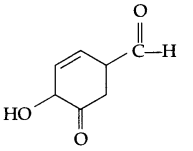(b) Explain the mechanism of nucleophilic addition reaction for a carbonyl compound.
(c) There are two -NH2 groups in semicarbazide, however, only one is involved in the formation of semicarbazone. Explain.
(d) Out of phenol and carboxylic acids which is more acidic and why?
(e) Give chemical reaction for Ketal formation.
OR
Fill in the blanks: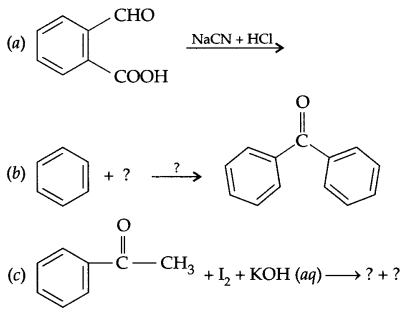(d) Arrange according to the increase in the given property:

1. HCOOH, CH3COOH, C6H5COOH (Acidic strength)
2. Butanal, Butanone & Butan-1 ol (Boiling point)

Question 25.
(a)

1. XeO2F2 + H2O →
2. NaOH + Br2
3. Zn3P2 + H2O →

(b) Draw shapes of XeF+5 & ICl+4

OR
Give reason for the following:

1. Noble gases are mostly inert.
2. Halogens are strong oxidising agents.
3. F2 is more reactive than Cl2.
4. Phosphorous shows greater tendency for catenation than nitrogen.
5. SeF6 easily get hydrolysed as compared to SF6.

Question 26.
(a) Thermal decomposition of methanoic acid is a first order reaction with a rate constant of 2.4 × 10-1 s-1 at a certain temperature. Calculate how long will it take for three fourth of the initial quantity of it to decompose.
(b) Write the order of reaction for the following reactions if K is given as

1. 2 × 10-4 s-1
2. 3.8 × 10-2 mol dm-3 s-1

OR
(a) The rate constant for the first order decomposition of H2O2 is given by the following equation:
log K = 14.34 – 1.25 × 104 K/T
Calculate Ea for this reaction and at what temperature will its half life period be 256 minutes?
(b) Mention the factors that affect the rate of a chemical reaction.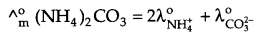= 2 × 60 + 80 = 200 S cm2 mol-1

Adsorption is a phenomenon of attracting and retaining the molecules of a substance on the surface of a liquid or solid, leading to higher concentration on the surface as compared to bulk. Such force of attraction leads to the release in energy as well as the decrease in kinetic energy of free molecules. As a result adsorption is an exothermic process.

Ce4+.

Iron metal in the form of iron scraps.

[Pt(NH3)2(CH3NH2)(CO)2]Cl4.

For 100 molecules of Fe0.93 O1.00 there will be 93 Fe atoms and 100 oxygen atoms
If Fe2+ ions are x then
Fe3+ ions = 93 – x
Total charge on x ions of Fe2+ and (93 – x) ions of Fe3+ will be equal to 200, i.e. the charge on 100 O2- ions.
Hence 2x + 3(93 – x) = 200
x = 79, i.e. Fe2+ ions = 79 and Fe3+ ions = 93 – 79 = 14.
% of Fe2+ ion = $$\frac { 79 }{ 93 }$$ × 100 = 85%
% of Fe3+ ion = $$\frac { 14 }{ 93 }$$ × 100 = 15%

1. Fe(OH)3 sol possesses positive charge on the dispersed phase, which is neutralised by the SO2-4 ions of K2SO4 and as a result coagulation takes place.
2. River water is a colloidal solution of various types of soil, clay, silt which gets coagulated by the electrolytes (various salts) present in sea water. As a result, silt get deposited at the meeting point of river water and sea in the form of delta.

1. Copper matte consists of Cu2S and FeS, which on oxidation in the presence of hot air converts FeS to FeO, which combines with silica, SiO2 to form the slag (FeSiO3).
2FeS + 3O2 → 2FeO + 2SO2 ↑
FeO + SiO2 → FeSiO3
2. Role of cryolite:
• It lowers the melting point of the mixture (Al2O3 + Na3AlF6) to about 1140 K.
• It increases the electrical conductivity of the mixture (as compared to the less ionisable Al2O3).

OR
Reactions taking place at higher temperature zone (900-1500 K):

• CaCO3 $$\underrightarrow { heat }$$ CaO + CO2
• C + CO2 → 2CO
• FeO + CO → Fe + CO2
• CaO + SiO2 → CaSiO3

Reactions taking place at lower temperature zone (500 – 800 K):

• Fe2O3 + CO → Fe3O4 + Fe3O4 + CO2
• Fe3O4 + CO → Fe + CO2
• Fe2O3 + CO → FeO + CO2

1. 2MnO4 + 5H2S + 6H+ → 2Mn2+ + 5S + 8H2O
2. Cr2O2-7 + 3NO2 + 8H+ → 2Cr3+ + 3NO3 + 4H2O

In analytical chemistry co-ordination compounds play an important role in both qualitative as well as quantitative analysis:

1. Qualitative analysis : Detection of Cu2+ ions by addition of NH3 to form deep blue complex.
Quantitative analysis : EDTA is used to find the hardness of water (e.g. metal ions like Ca2+, Mg2+, Zn2+ Fe2+ etc. can be estimated).
2. Medicinal chemistry :
• Cis platin → [Pt(NH3)2 Cl2] is used for the treatment of cancer.
• EDTA for complexing with Pb during lead poisoning.

1. Frenkel defect : It occurs in ionic compounds when there is large difference in the size of cation and anion, smaller ion occupies (get displaced) the interstitial space. In this defect, density of solid and formula does not get changed.
2. Ferrimagnetism : It is observed when the magnetic moments of the domains in the substance are aligned in parallel and antiparallel direction in unequal numbers. These substances are weakly attracted towards the magnetic field.
3. Paramagnetism : Such substances are weakly attracted by a magnet and possesses unpaired electrons. This phenomena of being attracted by magnetic field due to the presence of unpaired electrons is called paramagnetism.

1. Reverse osmosis : It is the movement of solvent particles from higher concentration of a solution to lower concentration of the solution through a semi-permeable membrane.
2. Peeled egg will start shrinking due to osmosis of water present in the egg that will come out through its membrane.
3. Coolant (ethylene glycol) needs to be added in car radiators to rim the vehicles at higher temperature by elevation of boiling point and in case of hill stations by depression in freezing point that prevents water from freezing

From question, I = 5 amp, mass of Na = 1.15 g, t = ?
Mass of Zn and Al needs to be calculated from Faraday’s second law.m = ZIt where Z = $$\frac { M }{ nF }$$ (Z = Chemical equivalence)
(m = Molar mass, n = change in No. of electrons)
so m = $$\frac { MIt }{ nF }$$, 1.15 = $$\frac { 23\times 5\times t }{ 1\times 6500 }$$
t = 965 seconds = 16.08 minutes.

From question:OR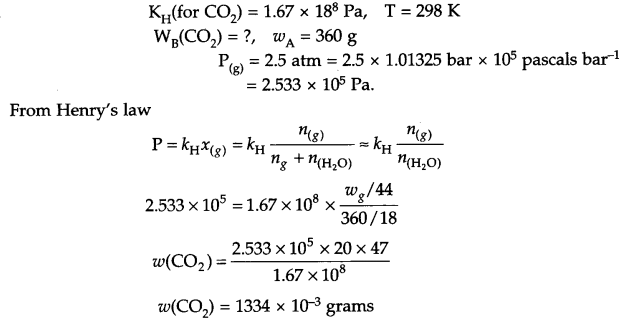1. Transition metals exhibit variable oxidation state and hence acts as good catalyst.
2. In case of zinc there is no impaired electrons in its valence shell as well as its size is large, so its enthalpy of atomisation is minimum.
3. In Ti4+, it has noble gas electronic configuration, so +2, and +3 state posses 3d2 or 3d1 configuration which is unstable and +4 oxidation state is quite stable.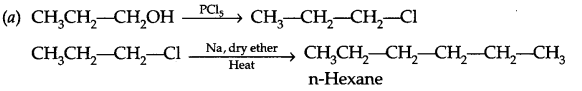(b) The nucleophile having two nucleophilic centres but at time only one get attached with electrophilic centre. For example -CN can attach through ‘C’ as well as N.
(c) p-dichrobenzene has symmetrical arrangement, hence closer packing of such molecules is possible in solid state as compared to ortho and meta isomer. As a result its melting is higher.1. Amino acids consists of amine group as well as carboxylic group in the same compound, as a result it will be amphoteric in nature.2. Thyonine
3. HI

(a) Nylon-6 is an example of condensation homopolymer, its monomer is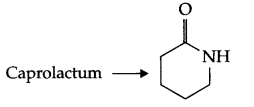(b) Monomers of PHBV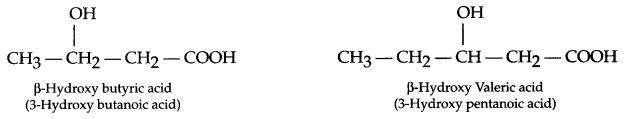(c) Buna-N or Buna-S

1. Antiseptic (imparts antiseptic property in soaps)
2. Analgesic (steroid-habit forming analgesic)
3. Tranquilizer (Antidepressant drug)

(a) (i)

 Reagent used Anisole Phenol Neutral FeCl3 No dark colour complex formation Forms dark coloured (Violet grey complex)

(ii)

 Reagent Propan-1-ol Ethanol I2 + NaOH + heat Yellow ppt. does not form Gives yellow ppt. of CHl3

(b) The OH group present on benzene ring activates it for SE (substitution, electrophilic) reaction at ortho and para positions due to the following resonance structures, as a result ortho and para substituted nitrophenol will form.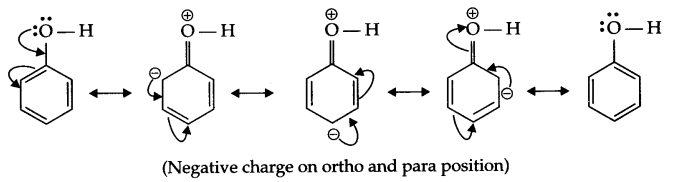(a) pKb of aniline is more, it means it is a weaker base than methyl amine.
In case of aniline due to resonance nitrogen acquires positive charge, hence donation of electron is less likely. Secondly, phenyl group is an electron withdrawing group while methyl group is an electron donating group.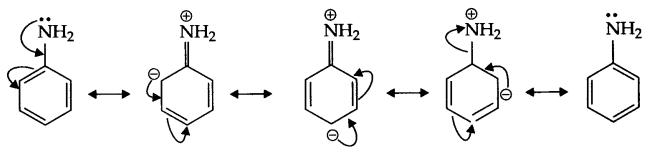(b) In H2O, methyl amine gives OH ions which reacts with FeCl3 to give hydrated ferric oxide.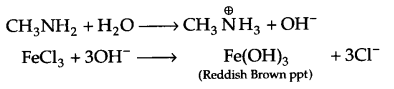(c) There is partial double bond character in halobenzene, hence it cannot be broken down easily and that is why aromatic amines cannot be prepared by Gabriel phthalimide synthesis.

1. CuFeS2
2. SO2
3. The released SO2 during this process causes environmental pollution. It is better if it can be used in the preparation of H2SO4.
4. Knowledge of science, concern for environment as well as businessman’s thinking:

(a) 4-Hydroxy-3-oxo cyclohex-5-ene carbaldehyde
(b) Example of a Nucleophilic addition reaction :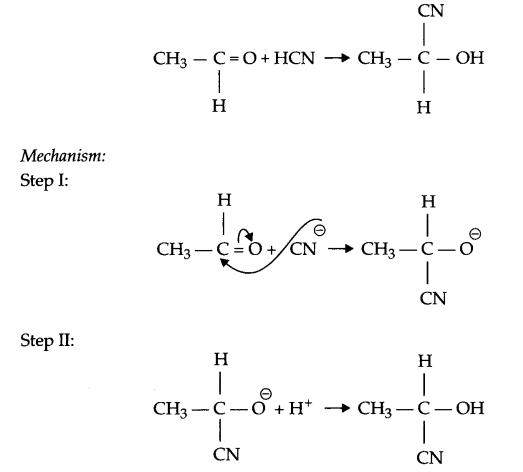(c) The lone pair of electrons on NH2 group attached to the carbonyl group is involved in resonance and hence is not available for the reaction.(d) Carboxylic acids are more acidic than phenol, reason being is resonance stabilised structure of carboxylic acids in which negative charge lies on ‘O’ atom. On the other hand, in phenol the negative charge lies on more positive carbon atom, hence contribution of such resonance structures is negligible.

(e) When ketone reacts with two moles of alcohol in the presence of HCl (g) ketal is formed.OR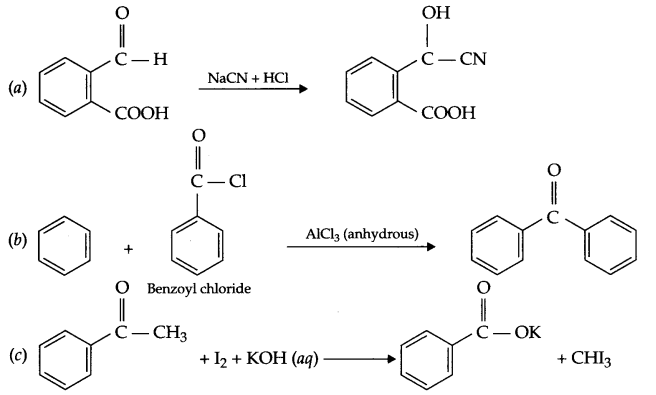(d)

1. C6H5COOH < CH3COOH < HCOOH
2. Butanone < Butanal < Butan-1-ol

(a)

1. XeO2F2 + 2H2O → XeO3 + 2HF
2. NaOH + Br2 → NaBr + NaBrO3
3. Zn3P2 + 6H2O → 3Zn(OH)2 + 2PH3

(b)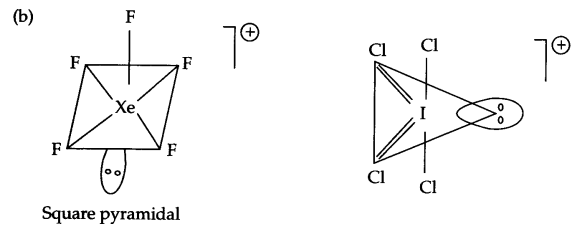OR

1. Noble gases possess completely filled octet electronic configuration and have high ionisation energy, hence they are mostly inert.
2. Halogens have high electron gain enthalpy and high reduction potential, so these are strong oxidising agents.
3. Bond dissociation enthalpy for F2 is much less than Cl2, so F2 is more reactive.
4. P-P single bond is more stronger than N-N single bond. There is lone pair-bond pair repulsions between two nitrogen atoms, hence there is a weaker single bond in phosphorous and much less catenation property.
5. S is sterically surrounded by 6 F atoms, hence cannot approach by lone pair of H2O, but due to larger size of selenium, hydrolysis is possible.

(a) From question K = 2.4 × 10-1 s-1
If [A]0 = 1 then [A]t =1 – 3/4 = $$\frac { 1 }{ 4 }$$
For first order reaction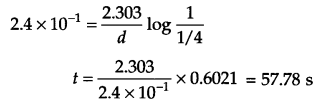(b)

1. first order
2. Zero order

OR
(a) From Arrhenius equation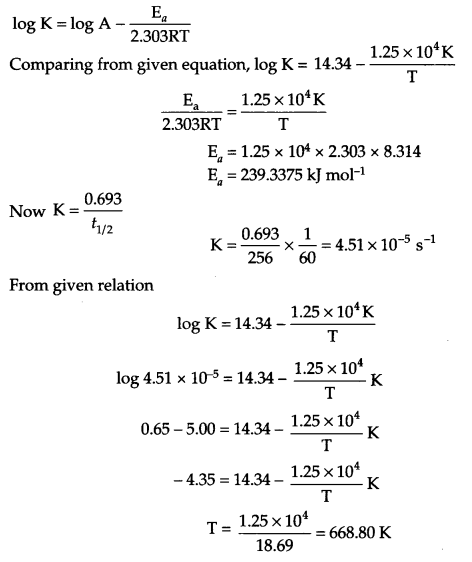(b)

• Concentration
• Temperature
• Pressure
• Catalyst

We hope the CBSE Sample Papers for Class 12 Chemistry Paper 5 help you. If you have any query regarding CBSE Sample Papers for Class 12 Chemistry Paper 5, drop a comment below and we will get back to you at the earliest.